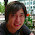## Wednesday, January 2, 2013

### Building a Gesture Recognizer - Part 2 Hidden Markov Models

In the last post on gesture recognition, we used a simple instance based recognizer. The distance measure we used is Dynamic Time Warping. This distance accounts for warping in the gesture.

A more prominent and realistic recognizer is using Hidden Markov Models.
Many Gesture and Speech or Handwritten recognition systems use these models.

A Hidden Markov Model is a probabilistic model. As Dynamic Time Warping, it models
time series while accounting for warping in the gesture.
In order to get an intuition on what a Hidden Markov Model is and how it works I will give
a brief introduction. I will not go over the math behind it since other people did a very good job at it .
A Hidden Markov Model is a probabilistic state machine. Each state has two probability distributions.
A continues one that models the data and a discrete one modeling the probability of transitioning
from a state to another. The continues one is called observation probability since it models the observed data. The probability distribution is a gaussian centered around a mean that discribes the data under that state best. For example in the image below we can see a three state Hidden Markov Model.
The time series has clearly three distinct parts. We model each part as one state, while the mean of the
state is the mean estimated from that part. The goal is to find the maximum likelihood state sequence
given the data and the model. We can infer which frame of the time series belongs to which
state using the observation probabilities and the transition probabilities.

How to perform that inference is described in the Rabiner paper on Hidden Markov Models on page 263 "Solution to Problem 2". The procedure's name is "Viterbi decoding". Once we have the state sequence we can calculate the probability of the time series being generated by a particular model.

For a gesture recognizer we build multiple of these models, one for each gesture. Then we collect a training set and use it to estimate the parameters of that model. During recognition we simply pick the model describing the data best.

In most cases Hidden Markov Models outperform Dynamic Time Warping solutions in accuracy and
speed. If you want to build a gesture recognizer using Hidden Markov Models you can use GART or
GT2K built by the research group I am currently working in. Both are build on the Hidden Markov Model Toolkit 3 (HTK3) so you have to install it first. I also recommend reading the HTK3 Book if you are interested in Hidden Markov Models in general. GT2K is a collection of shell scripts wrapping
HTK and it can be downloaded from the Contextual Computing Group. GART is a Java wrapper which is downloadable at the Contextual Computing Group, too.

 Lawrence Rabiner: "A Tutorial on Hidden Markov Models and Selected Applications in Speech Recognition", Proceedings of the IEEE Vol 77, No 2, 1989

1.hi!
its a really nice posting. thank you
i have some questions about HMM and DTW, so
if you dont mind, can i email you to ask some
questions?
thank you!

1.Sure, you can email me ... happy you liked it :D

2.